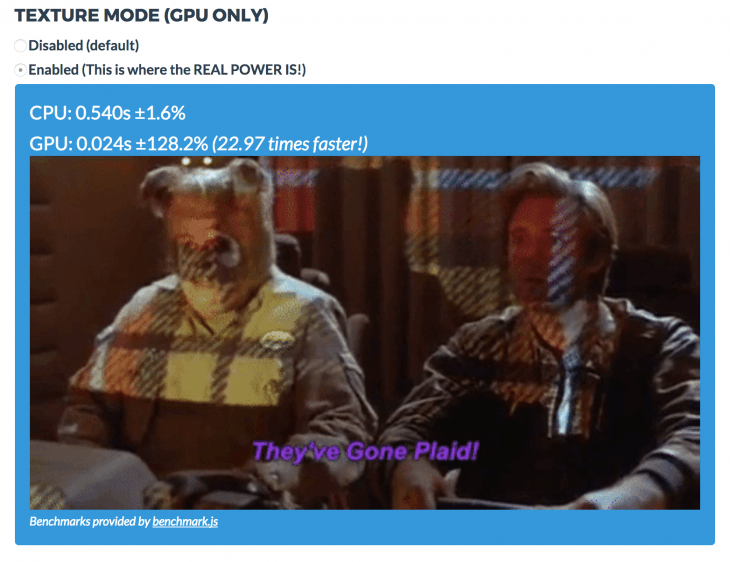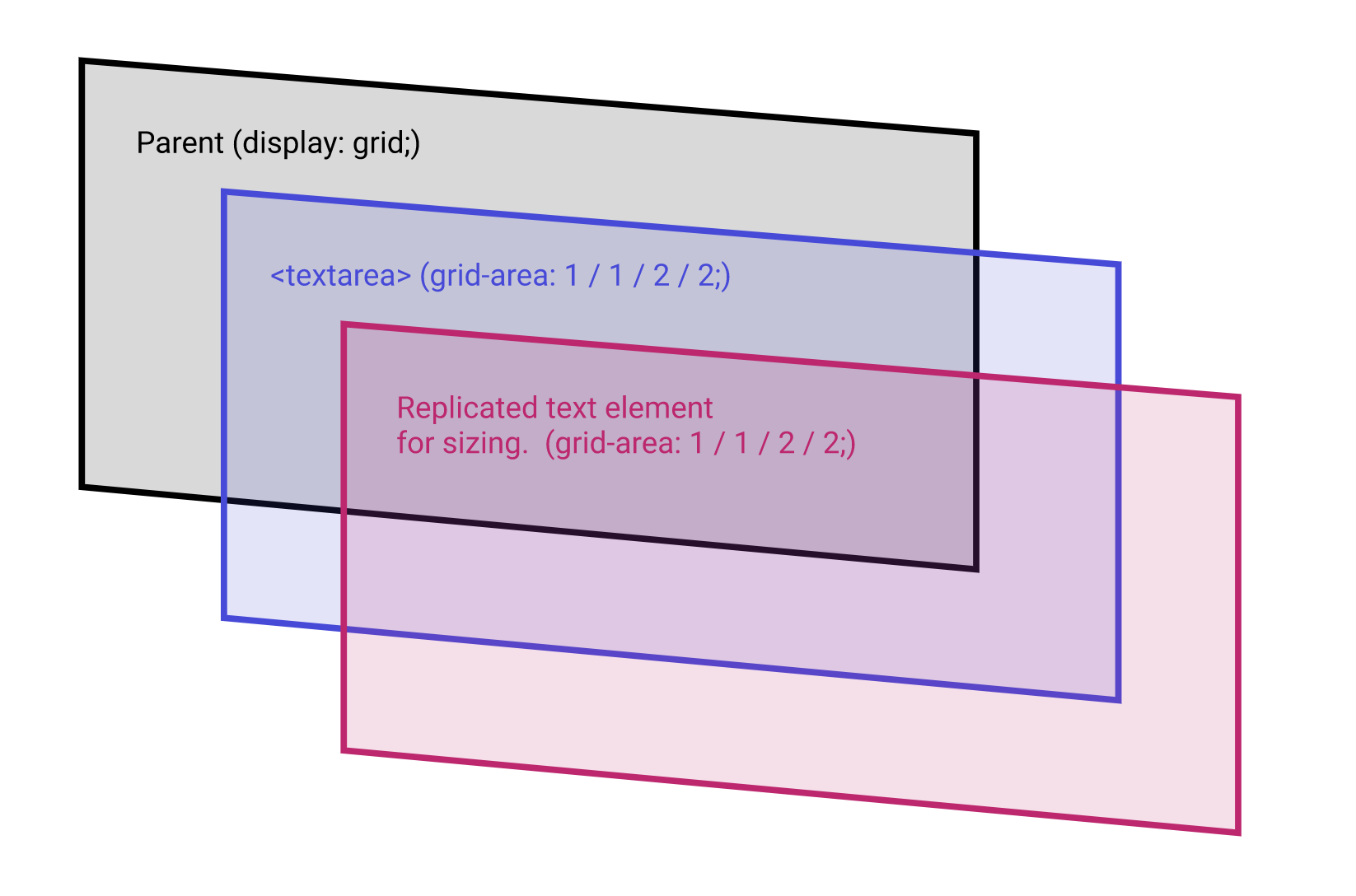# 使用GPU.js改善JavaScript性能## 什么是 GPU.js？

GPU.js 是一个针对 Web 和 Node.js 构建的 JavaScript 加速库，用于在图形处理单元（GPGPU）上进行通用编程，它使你可以将复杂且耗时的计算移交给 GPU 而不是 CPU，以实现更快的计算和操作。还有一个备用选项：在系统上没有 GPU 的情况下，这些功能仍将在常规 JavaScript 引擎上运行。

## 为什么要使用 GPU.js

• GPU 可用于执行大规模并行 GPGPU 计算。这是需要异步完成的计算类型
• 当系统中没有 GPU 时，它会优雅地退回到 JavaScript
• GPU 当前在浏览器和 Node.js 上运行，非常适合通过大量计算来加速网站
• GPU.js 是在考虑 JavaScript 的情况下构建的，因此这些功能均使用合法的 JavaScript 语法## GPU.js 的工作方式

`gpu.createKernel` 方法创建了一个从 JavaScript 函数移植过来的 GPU 加速内核。

## GPU.js 入门

``````sudo apt install mesa-common-dev libxi-dev  // using Linux
``````

npm

``````npm install gpu.js --save
// OR
yarn add gpu.js
``````

``````import { GPU } from ('gpu.js')

// OR
const { GPU } = require('gpu.js')

const gpu = new GPU();
``````

### 乘法演示

``````const getArrayValues = () => {

// 在此处创建2D arrary
const values = [[], []]

// 将值插入第一个数组
for (let y = 0; y < 600; y++){
values.push([])
values.push([])

// 将值插入第二个数组
for (let x = 0; x < 600; x++){
values\[0\][y].push(Math.random())
values\[1\][y].push(Math.random())
}
}

// 返回填充数组
return values
}
``````

``````const gpu = new GPU();

// 使用 `createKernel()` 方法将数组相乘
const multiplyLargeValues = gpu
.createKernel(function(a, b) {
let sum = 0;
for (let i = 0; i < 600; i++) {
sum +=
aaaaaaaaaaaaaaaa\[this.thread.yyyyyyyyyyyyyyyy\][
i
] *
bbbbbbbbbbbbbbbb\[iiiiiiiiiiiiiiii\][this.thread.x];
}
return sum;
})
.setOutput([600, 600]);
``````

``````const largeArray = getArrayValues();
const out = multiplyLargeValues(
largeArray,
largeArray
);
``````

``````console.log(out\[y\][x]) // 将元素记录在数组的第x行和第y列
console.log(out\[10\]) // 记录输出数组第10行和第12列的元素
``````

## 运行 GPU 基准测试

``````npm install @gpujs/benchmark

const benchmark = require('@gpujs/benchmark')

const benchmarks = benchmark.benchmark(options);
``````

`options` 对象包含可以传递给基准的各种配置。

## 结束

上一篇自动增长Textareas的最干净技巧

2020-12-02初学者的技术写作：技术博客基础知识A-Z指南

2020-11-29
目录